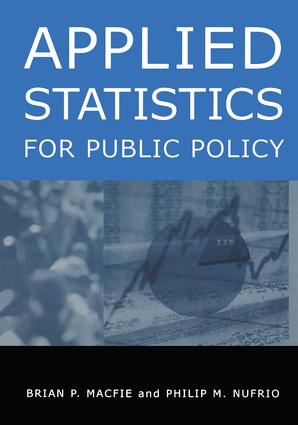# Applied Statistics for Public Policy

## 1st Edition

Routledge

538 pages

##### Purchasing Options:\$ = USD
Hardback: 9780765612397
pub: 2005-06-30
SAVE ~\$29.00
\$145.00
\$116.00
x
eBook (VitalSource) : 9781315669243
pub: 2017-07-28
from \$28.98

FREE Standard Shipping!

### Description

This practical text provides students with the statistical tools needed to analyze data, and shows how statistics can be used as a tool in making informed, intelligent policy decisions. The authors' approach helps students learn what statistical measures mean and focus on interpreting results, as opposed to memorizing and applying dozens of statistical formulae. The book includes more than 500 end-of-chapter problems, solvable with the easy-to-use Excel spreadsheet application developed by the authors. This template allows students to enter numbers into the appropriate sheet, sit back, and analyze the data. This comprehensive, hands-on textbook requires only a background in high school algebra and has been thoroughly classroom-tested in both undergraduate and graduate level courses. No prior expertise with Excel is required. A disk with the Excel template and the data sets is included with the book, and solutions to the end-of-chapter problems will be provided on the M.E. Sharpe website.

### Table of Contents

Preface; Unit I. Descriptive Statistics; 1. Introduction: What Is Statistics All About? 2. Using Polystat to Do Statistical Analysis; 3. Presentation of Data; 4. Summarizing Data and Using Descriptive Statistics; Unit II. Basic Probability and Probability Distributions; 5. Basic Probability: Theory and Applications; 6. Sampling and the Normal Distribution; 7. The Central Limit Theorem; Unit III. Hypothesis Testing; 8. Introduction to Inferential Statistics; 9. Estimating Population Means, Proportions, and Sample Size with Confidence; 10. Validating a Hypothesis About a Single Population Mean Using a Sample; 11. Validating Hypotheses Between Two Population Means; 12. Validating Hypotheses About a Single Population Proportion; 13. Validating Hypotheses About Two Population Proportions; Unit IV. Measures of Association; 14. Comparing More than Two Population Means with Anova; 15. Comparing More than Two Population Proportions Using the Chi-Square Test; 16. Determining Relationships for Two Variables Using Simple Correlation; 17. Measuring Relationships Between Two Variables with Simple Regression Analysis; 18. Measuring Multivariate Relationships with Multiple Regression Analysis; 19. Planning Statistical Research; Appendix; Key Formulas

### Subject Categories

##### BISAC Subject Codes/Headings:
BUS091000
BUSINESS & ECONOMICS / Business Mathematics
POL000000
POLITICAL SCIENCE / General